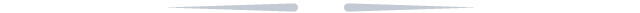# 【进阶篇】命令行参数使用案例

2018/02/06 15:37

1

--use_gpu=1/0 \

#1:GPU,0:CPU(默认为1)

--config=network_config \

--save_dir=output \

--trainer_count=COUNT \

#(默认为1)

--test_period=M \

#(默认为0)

--num_passes=N \

#(默认为100)

--log_period=K \

#(默认为100)

--dot_period=1000 \

#(默认为1)

#[--show_parameter_stats_period=100] \

#(默认为0)

#[--saving_period_by_batches=200] \

#(默认为0)

1) 将命令参数传给网络配置

config_args是一个很有用的参数，用于将参数传递给网络配置：

--config_args=generating=1,beam_size=5,

layer_num=10 \

get_config_arg可用于在网络配置中解析这些参数，如下所示：

generating = get_config_arg('generating', bool, False)

beam_size = get_config_arg('beam_size',int, 3)

layer_num = get_config_arg('layer_num', int, 8)

get_config_arg：

get_config_arg(name, type, default_value)

---name: --config_args中指定的名字

---type: 值类型，包括bool, int, str, float等

---default_value: 默认值

2) 使用模型初始化网络

--init_model_path=model_path

2

paddle train --job=test \

--use_gpu=1/0

--config=network_config \

--trainer_count=COUNT \

--init_model_path=model_path \

---使用init_model_path指定测试的模型

---只能测试单个模型

paddle train --job=test \

--use_gpu=1/0

--config=network_config \

--trainer_count=COUNT \

--model_list=model.list \

---使用model_list指定测试的模型列表

---可以测试多个模型，文件model.list如下所示：

./alexnet_pass1

./alexnet_pass2

paddle train --job=test \

--use_gpu=1/0 \

--config=network_config \

--trainer_count=COUNT \

--save_dir=model \

--test_pass=M \

--num_passes=N \

3

1) 本地训练

2) 集群训练

=True。请参照网络配置的文档了解更详细的信息

--ports_num_for_sparse=1

#(默认为0)

4

parallel_nn

--parallel_nn=true

#command line:

paddle train --use_gpu=true --parallel_nn=true trainer_count=COUNT

default_device(0)

fc1=fc_layer(...)

fc2=fc_layer(...)

fc3=fc_layer(...,layer_attr=ExtraAttr(device=-1))

default_device(0): 设置默认设备号为0。这意味着除了指定device=-1的层之外，其他所有层都会使用GPU计算，每层使用的GPU号依赖于参数trainer_count和gpu_id(默认为0)。在此，fc1和fc2层在GPU上计算

device=-1: fc3层使用CPU计算

trainer_count:

---trainer_count=1: 如果未设置gpu_id，那么fc1和fc2层将会使用第1个GPU来计算。否则使用gpu_id指定的GPU

---trainer_count>1: 在trainer_count个GPU上使用数据并行来计算某一层。例如，trainer_count=2意味着0号和1号GPU将会使用数据并行来计算fc1和fc2层

#command line:

paddle train --use_gpu=true --parallel_nn=true --trainer_count=COUNT

#network:

fc2=fc_layer(input=l1, layer_attr=ExtraAttr(device=0), ...)

fc3=fc_layer(input=l1, layer_attr=ExtraAttr(device=1), ...)

fc4=fc_layer(input=fc2, layer_attr=ExtraAttr(device=-1), ...)

trainer_count=1:

---使用0号GPU计算fc2层

---使用1号GPU计算fc3层

---使用CPU计算fc4层

trainer_count=2:

---使用0号和1号GPU计算fc2层。

---使用2号和3号GPU计算fc3层。

---使用CPU两线程计算fc4层。

trainer_count=4:

---运行失败（注意到我们已经假设机器上有4个GPU），因为参数allow_only_one_model_on_one_gpu默认设置为真

device!=-1时设备ID号的分配：

(deviceId + gpu_id + threadId * numLogicalDevices_) % numDevices_

deviceId:              在层中指定

gpu_id:                 默认为0

threadId:              线程ID号，范围: 0,1,..., trainer_count-1

numDevices_:      机器的设备(GPU)数目

numLogicalDevices_:   min(max(deviceId + 1), numDevices_)*原创贴，版权所有，未经许可，禁止转载

*欢迎在留言区分享您的观点### 作者的其它热门文章

0
0 收藏

0 评论
0 收藏
0